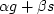Next: Magic Up: ITERATIVE METHODS Previous: Why steepest descent is

In the conjugate-gradient method, not a line, but rather a plane, is searched. A plane is made from an arbitrary linear combination of two vectors. One vector will be chosen to be the gradient vector, say g. The other vector will be chosen to be the previous descent step vector, say s = xj - xj-1. Instead ofwe need a linear combination, say.For minimizing quadratic functions the plane search requires only the solution of a two-by-two set of linear equations forand.The equations will be specified here along with the program. (For nonquadratic functions a plane search is considered intractable, whereas a line search proceeds by bisection.)